Study Guide

# Thermodynamics Questions

## Thermodynamics Questions

1. He-man wants to build a play structure for his little sister's birthday. He just learned that heat causes matter to expand. Similarly, matter contracts when it is cool. He's a bit worried that the play structure might buckle under the extreme heat differences in the back yard, since even superheroes can't change the weather.

Wood has a specific heat capacity of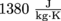and Steel has a specific heat capacity of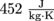. Assuming he would use the same mass of either building material, which material should he choose to minimize the amount of buckling?

2. A scuba diver takes a deep breath of air from her tank, filling her lungs completely while 15 meters below the surface of the ocean. When the diver ascends, the temperature of air in her lungs does not change. Why is it a very, very bad idea for the diver to surface very rapidly when her lungs are full of air?

3. Why is it impossible to ever build a heat engine that has a Carnot efficiency of 1?

4. Pressure cookers are fancy pots that cook food under high pressure. Once the pot is pressurized, it takes very little flame to cook food in a much shorter amount of time than in a normal, non-pressurized thermal pot. If we assume that food is cooked by steam, why does this pot need so much less energy to cook food?

5. Mary wants to heat a 10 cm long, 10 g glass rod that has been sitting in a refrigerator at 4 °C, so that it increases in length by a measurable amount. We don't know why; there's just something about Mary, and we've stopped questioning why she does stuff like this.

The coefficient of linear thermal expansion of glass is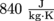and the specific heat capacity of glass is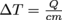. Assuming that she can see a 0.1 cm increase in length, how much heat should she add to the glass rod?

6. Don't try this at home! Using what we learned in this chapter, why is it a bad idea to heat a can of hairspray?

7. Two friends are at the state fair. One has a mole of puppies and the other possesses a mole of carbon. Which should enter a "whose mole weighs more" contest and which a "whose mole contains more individuals" contest?

8. Napolean Dynamite succumbed to his inner dork and entered a thermodynamic cycle race. The cycle that does the most work wins. He picked a thermodynamic cycle but needs to decide whether he wants to run it in a forward direction (A → B → C → A) or in the reverse direction (A → C → B → A). Which should he pick?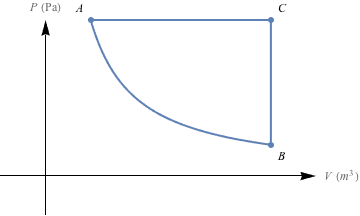9. Why is boiling water vigorously to cook pasta (as opposed to simmering) a waste of energy unless the goal is to produce more steam more quickly?

10. Channeling our inner preschooler, we want to pop a balloon. We can either fill it with helium or with air. Whichever one we choose, we'll add the same total number of molecules and they will have the same average velocity. The volume of the blown up balloon will be the same in both cases. The mass of a helium atom is 4 × 10-3 kg. Air is over 80% nitrogen, so to make things simpler, let's assume that we're adding only nitrogen atoms. The mass of a nitrogen atom is 1.4 × 10-2 kg. Which balloon is more likely to pop?

### Solutions

1. To minimize the amount of buckling, we need to minimize how much the temperature of the material changes for a set amount of heat being applied. That means we need to use the equation Q = cmΔT. The only difference between the two materials is the specific heat of each. We need the material that has the smallest ΔT per unit of heat added. Since, we want the specific heat capacity, c, to be as large as possible to minimize the change in temperature, and that means a play structure of wood.

2. This is an application of Boyle's law. When temperature is constant, P1V1 = P2V2. The pressure on the air in the diver's lungs 15 meters below the surface is much higher than the pressure at the surface of the ocean, so P1 > P2. When the diver ascends very quickly, the volume of air in her lungs expands to keep P1V1 equal to P2V2.

The air in her lungs expands and could burst her lungs, sinuses, and ears. We hear that little factoid, and we say NOPE. This is why divers ascend slowly and make sure to not hold their breath. As the diver ascends, she wants to make sure that she allows air to escape from her lungs as the air's volume increases.

3. The Carnot efficiency is given by the equation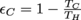. For εC to be 1,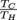would have to equal zero. This only happens when TC equals 0. Since we measure temperature in Kelvin for this equation, the temperature of the cold reservoir would have to be absolute zero, which, haha, good luck with that.

4. In a pressure cooker, the total volume stays the same, so that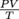is constant. As pressure increases in the pressure cooker, then, the temperature, T, increases. For a normal, non-sealed pot, all the increase in temperature to cook food comes from the flame itself, and not from any thermodynamic magic. When we cook our food with a pressure cooker, the food is heated both by the stove flame and by the increase in pressure in the pot, which takes less flame (and less time!) to cook up a scrumptious meal.

5. First let's calculate how much the temperature of the rod must increase to change the length by 0.1 cm:Then we calculate how much heat is required to change the temperature of the glass rod by 3030 °C:She should heat the rod by a blistering 25,452 joules.

6. A can of hairspray is essentially a fixed volume of gas in a container whose volume can't expand. In other words, this is another example where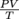is constant. If you raise the temperature by heating the hairspray can, the volume of the gas will try to increase, because the hairspray gas molecules are increasing their average kinetic energy. Those hard aluminum walls aren't budging, though, so the pressure of the gas on the can increases instead. If we heat the hairspray too much, eventually the pressure is so high that it bursts the can holding the hairspray—kaboom! That's why it's a bad idea.

7. The first definitely should enter the weight contest. A mole of puppies contains exactly the same number of individuals (6.022 × 1023) as a mole of carbon, but each puppy weighs a smidge more than an atom of carbon. No one would win a "whose mole contains more individuals" contest, because a mole is a mole is a mole, no matter what. It's a unit of measure that's always equal to 6.022 × 1022 of whatever we're dealing with.

8. The amount of work done for each step is equal to the area under the PV diagram. Let's write down whether work is done by or on the system for each step.

Forward cycle:

Step AB: work is done by the system, W = negative
Step BC: no work is done, W = 0
Step CA: work is done on the system, W = positive

The area under CA is larger than the area under AB, so the total amount of work done by this forward cycle is positive. In this case, we're putting more work into the system than we're getting out of it.

Reverse cycle:

Step AC: work is done by the system, W = negative
Step CB: no work is done, W = 0
Step BA: work is done on the system, W = positive

The area under AC is greater than the area under BA, so the total amount of work done by the reverse cycle is negative. We're getting out more work than we put in.

Napolean Dynamite would definitely want to run this cycle in reverse for his thermodynamic cycle race. With him participating, we imagine such a thing might actually exist.

9. When matter experiences a phase change, the temperature of matter doesn't change. All of that extra energy that we're adding to boil the water vigorously goes into turning the water into steam, not into raising the temperature of the water. To be fair, though, a vigorously boiling pot has a more uniform 100 ˚C temperature than a simmering one, since a boiling pot isn't a closed system: it loses energy to the air and through the sides of the pot.

10. The pressure that the gas inside of a balloon exerts on the balloon walls makes balloons pop. The formula to calculate the amount of pressure on a gas is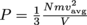. Since V, N, and vavg are the same in both cases, only the mass, m, is different for helium versus an air balloon. Since the mass of nitrogen is higher than the mass of helium, the air balloon is more likely to pop. Don't let the 4 vs. 1.4 at the beginning of each measure fool you; we've got to check exponents on the scientific notation at the end, too.